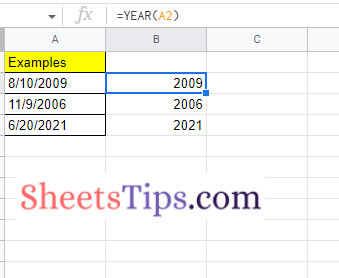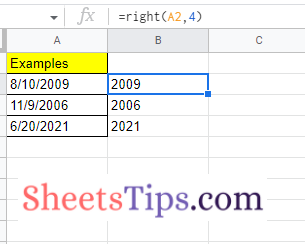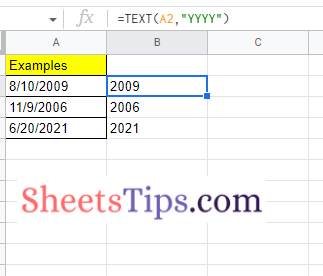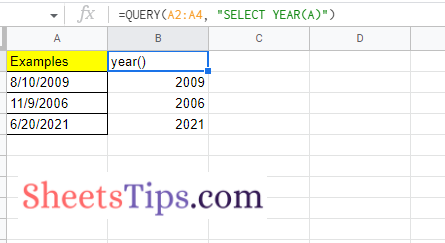# How to Extract the Year from Google Sheets-YEAR Function in Google Sheets

There are several functions in Google Sheets that help you perform various actions and operations without any difficulty. Of all the functions, Google Sheets has one of the most useful functions named YEAR. Using the YEAR function in Google Sheets, we can simply extract the year based on the date. To simplify the dataset based on the dates, we can use the YEAR function. For example, let us say you want to know the list of employees who have completed 5 years in your organization. To know the same, you can extract the year of joining just by using the YEAR Function.

Interesting, isn’t it?

This is an example. With the help of the YEAR function, we can do many more things. But it is extremely important for one to know how to extract the year for any given dataset. And hence, here is an article that talks everything about how to extract a year in the spreadsheet using Google Sheets Tips. Read further to find out more.

## Syntax of the YEAR Function in Google Sheets

In order to use the YEAR function in Google Sheets, it is extremely important for one to know the syntax of the same and how to use it in Google Sheets. So let us first understand the syntax of the YEAR function in Google Sheets.

=YEAR (Date)

A date is a date value or a cell carrying a date from which the year value in YYYY format should be extracted. As an input to this function, you may also use functions that return a date or a number.

### How to Extract Years in Google Sheets Using the YEAR Function?

The steps to extract the year of any given dataset using the YEAR function are outlined below:

• 1st Step: Launch the Google Spreadsheet.
• 2nd Step: Now move to the date values from where you would like to extract the year.
• 3rd Step: Enter the formula “=Year(Cell Range)“. In this example, the cell range is A2. Hence my formula is =Year(A2).
• 4th Step: You will see the Year being extracted. Now drag the formula applied cell to the other parts of the cell to extract the year and you will see the results as shown in the image below.### How to Extract the Year from a Date using the RIGHT Function?

Follow the steps listed below to extract the year using the RIGHT function.

• 1st Step: Open the Google Spreadsheet on your device.
• 2nd Step: Move to the cell where you want to extract the year.
• 3rd Step: Type the formula “=right (A2,4)“.
• 4th Step: Press the “Return” key and you will find the results.In the RIGHT function, we are giving the command to extract the right-side characters of the cells. Following that, in the RIGHT function, we should also specify how many characters it should extract.

### How to Extract Year Using the TEXT Function in Google Sheets?

Apart from the above methods, the TEXT function is also one of the easiest methods which help us to extract the year in Google Sheets. The steps to extract the year from the given dates using the TEXT function are given below:

• 1st Step: Open the Google Sheets to extract the year.
• 2nd Step: Move to the cell from where you want to extract the year from the given dates.
• 3rd Step: Enter the formula “=TEXT(A2, YYYY)“. A2 refers to the cell range.
• 4th Step: Press the “Return” key and you will find the results as shown in the image given below.### How to Extract Year Using the Query Function?

We can also extract the year from the dates simply by using the QUERY function in Google Sheets. The steps to year extraction in a spreadsheet using the QUERY function are explained below.

• 1st Step: Open the Google Spreadsheet.
• 2nd Step: Move to the cell where you want to extract the year from the provided dates.
• 3rd Step: Enter the formula “=QUERY (A2:A4, “SELECT YEAR (A)”)“.
• 4th Step: Press the “Enter” key and you will fill in the results as shown in the image given below.